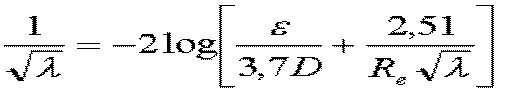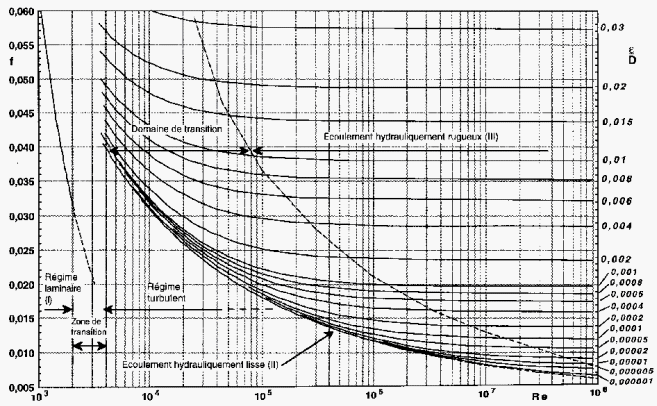the Colebrook-White formula is used to evaluate the coefficient of friction losses in the pipes (Major head loss and linear losses), for all values ​​of the Reynolds number (flow regime)Mecaflux uses this equation for Re > 105

Colebrook charts giving the major losses coefficient (left ordinate) as a function of Reynolds number (x-axis) and the relative roughness (right ordinate)